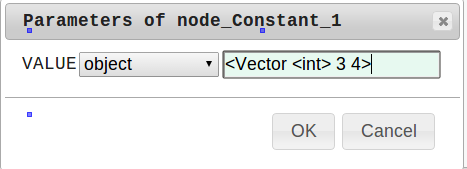## 4.2.1 Vector

Vector is the type that stores an array of data. Any data may be stored in Vector . Vector< Obj > , which has ObjectRef  as an element, and Vector< int >  and Vector< float > , which have values (int , float ) as elements are typically used. It is often used for value pairs, for example to designate a group of angles in ConstantLocalization  and to present a group of localization results, which are the output of LocalizeMUSIC . The following are definitions for the Vector  type. Base Vector   is the type for which methods in FlowDesigner  are implemented. As shown below, the Vector  type conforms to the vector  type of the STL.

template<class T> class Vector :
public BaseVector, public std::
vector<T>


The ToVect  node in the Conversion  category accepts inputs such as int  and float  and outputs a Vector  with only one value as an element. Moreover, when the user wishes to use Vector  as a parameter of a node, Object  should be used as the parameter type, which can be entered in text as shown below. For example, when the user wishes to enter a Vector  of int s that has two elements, 3 and 4, as the parameter, character strings are to be entered as shown in Figure 4.2. However, for character strings, note that Vector  must come next to the first character <  without any spaces.Figure 4.2: Input example of Vector